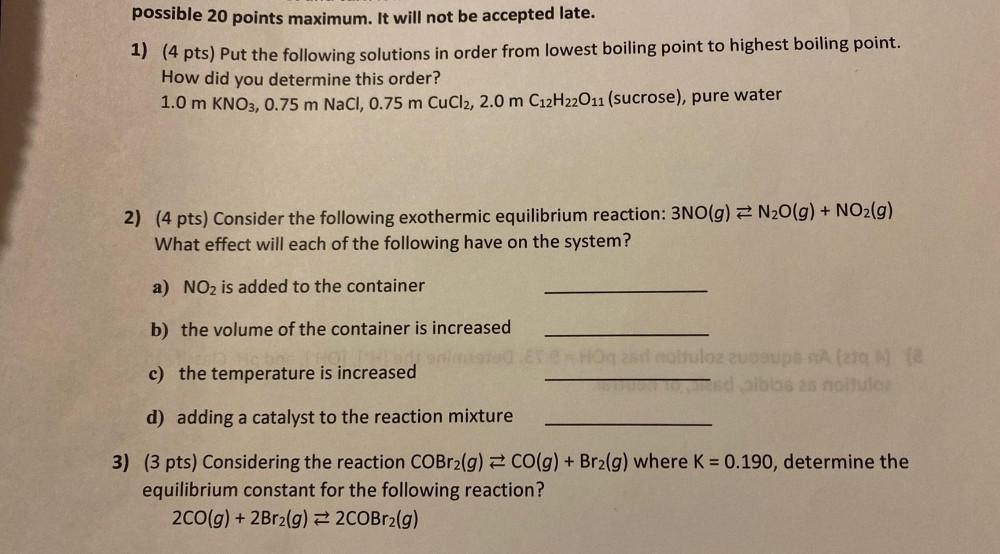Question:

# possible 20 points maximum. It will not be accepted late. 1) (4 pts) Put the following solutions in order from lowest boiling popossible 20 points maximum. It will not be accepted late. 1) (4 pts) Put the following solutions in order from lowest boiling point to highest boiling point. How did you determine this order? 1.0 m KNO3, 0.75 m NaCl, 0.75 m CuCl2, 2.0 m C12H22011 (sucrose), pure water 2) (4 pts) Consider the following exothermic equilibrium reaction: 3NO(g) = N2O(g) + NO2(g) What effect will each of the following have on the system? a) NO2 is added to the container b) the volume of the container is increased nimi c) the temperature is increased lozzuogu na sdibiosas no solo d) adding a catalyst to the reaction mixture 3) (3 pts) Considering the reaction COBr2(g) = CO(g) + Brz(g) where K = 0.190, determine the equilibrium constant for the following reaction? 200(g) + 2Br2(g) = 2COBr2(g)